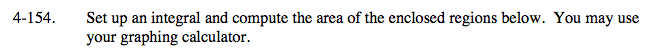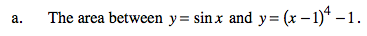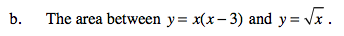### Home > CALC > Chapter Ch4 > Lesson 4.4.3 > Problem4-154

4-154.Find the bounds by computing the x-values of the points of intersection between the two functions.
sinx = (x −1)4 −1
a = 0, b = 2.163 (using a calculator)

Determine which is the upper function (the one with higher y-values) and which is the lower function (the one with lower y-values). If you are using a calculator: look at the graph. If not: Evaluate a point in between the bounds to see which function has the higher y-values.Refer to hints in part (a).